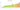# UZS

## Uzbekistan Som

### Uzbekistani som

The sum is Uzbekistan's national currency.

#### how has the value of the currency changed in the last year?## USD 1 = UZS 12,155

How much is10 US dollars worth inUzbekistani som?
At the current exchange rate, 10 US dollars is worth 121,551.37 Uzbekistani som
How much is50 US dollars worth inUzbekistani som?
At the current exchange rate, 50 US dollars is worth 607,756.86 Uzbekistani som
How much is100 US dollars worth inUzbekistani som?
At the current exchange rate, 100 US dollars is worth 1,215,513.72 Uzbekistani som
How much is500 US dollars worth inUzbekistani som?
At the current exchange rate, 500 US dollars is worth 6,077,568.58 Uzbekistani som
How much is2,000 US dollars worth inUzbekistani som?
At the current exchange rate, 2,000 US dollars is worth 24,310,274.33 Uzbekistani som
How much is10 US dollars worth inUzbekistani som?
At the current exchange rate, 10 US dollars is worth 121,551.37 Uzbekistani som
How much is50 US dollars worth inUzbekistani som?
At the current exchange rate, 50 US dollars is worth 607,756.86 Uzbekistani som
How much is100 US dollars worth inUzbekistani som?
At the current exchange rate, 100 US dollars is worth 1,215,513.72 Uzbekistani som
How much is500 US dollars worth inUzbekistani som?
At the current exchange rate, 500 US dollars is worth 6,077,568.58 Uzbekistani som
How much is2,000 US dollars worth inUzbekistani som?
At the current exchange rate, 2,000 US dollars is worth 24,310,274.33 Uzbekistani som